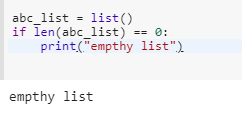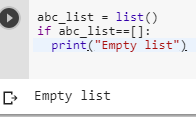3 views
in Python
edited

suppose I have passed : list=[]

How can I check if a list is empty or not?

by (10.9k points)
edited

@Anvi, following is an implicit way of checking if a list is empty or not:

if not list:

print("empty list")

by (106k points)
edited by

We have many ways to check whether a list is empty or not:-

The first way we can check is by using its length:-

abc_list = list()

if len(abc_list) == 0:

print(“Empty list”)The second way we can check by direct comparison whether the list is empty:-

abc_list = list()

if abc_list==[]:

print(“Empty list”)